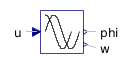Signal Pressure - MapleSim Help

Signal Pressure

Generic pressure source using the input signal as the source pressureDescription The Signal Pressure component generates a pressure across its ports proportional to an input signal.Equations $p={p}_{A}-{p}_{B}=\mathrm{inp}$ $q={q}_{A}=-{q}_{B}$Variables

 Name Units Description Modelica ID $p$ $\mathrm{Pa}$ Pressure across component p $q$ $\frac{{m}^{3}}{s}$ Flow rate through component qConnections

 Name Description Modelica ID $\mathrm{portA}$ Upstream hydraulic port portA $\mathrm{portB}$ Downstream hydraulic port portB $\mathrm{inp}$ Real input; pressure in $\mathrm{Pa}$ inp